## Physics

Question 1.
(a) Two stable isotopes of lithium 63Li and 37Li have respective abundances of 7.5% and 92.5%. These isotopes have masses 6.01512 u and 7.01600 u respectively. Find the atomic mass of lithium.
(b) Boron has two stable isotope.s, 105B and 115B Their respective masses are 10.01294 u and 11.00931 u, and the atomic mass of boron is
10.811 u. Find the abundances of 105B and 115B.
Solution:
Abundance of 63Li is 7.5% and abundance(b) Let abundance of 105B x% than abundance of 115B will be (100 – x)%.Question 2.
The three stable isotopes of neon : 2010Ne, 2110Ne and have respective abundances of 90.51%, 0.27% and 9.22%. The atomic masses of the three isotopes are 19.99 u, 20.99 u and 21.99 u, respectively. Obtain the average atomic mass of neon.
Solution:
Average atomic mass of neon with the given abundances,Question 3.
Obtain the binding energy (in MeV) of a nitrogen nucleus (147N), given m (147N) = 14.00307 u
Solution:
The nucleus contains 7 protons and

Question 4.
Obtain the binding energy of the nuclei 5626Fe and 20983Bi in units of MeV from the following data:
m(5626Fe) = 55.934939 u
m(20983Bi) = 208.980388 u
Solution:
Let us first find the binding energy ofQuestion 5.
A given coin has a mass of 3.0 g. Calculate the nuclear energy that would be required to separate all the neutrons and protons from each other. For simplicity assume that the
coin is entirely made of 6329Cu atoms (of mass 62.92960 u).
Solution:
Let us first find the B.E. of each copper nucleus and then we can find binding energyQuestion 6.
Write nuclear reaction equations for
(i) a-decay of 22688Ra
(ii) a-decay of 24294Pu
(iii) p-decay of 3215P
(iv) p-decay of 21083Bi
(v) p+-decay of 116C
(vi) p+-decay of 9743Tc
(vii) Electron capture of 12054Xe
Solution:Question 7.
A radioactive isotope has a half-life of T years. How long will it take the activity to reduce to
(a) 3.125%
(b) 1% of its original value?
Required time, as cannot be solved by direct calculation as in part (a).
Solution:

Required time, as cannot be solved by direct calculation as in part (a)Question 8.
The normal activity of living carbon-containing matter is found to be about 15 decays per minute for every gram of carbon. This activity arises from the small proportion of radioactive 146C present with the stable carbon isotope 126C. When the organism is dead, its interaction with the atmosphere (which maintains the above equilibrium activity) ceases and its activity begins to drop. From the known half-life (5730 years) of 146C, and the measured activity, the age of the specimen can be approximately estimated. This is the principle of 146C dating used in archaeology. Suppose a specimen from Mohenjodaro gives an activity of 9 decays per minute per gram of carbon. Estimate the approximate age of the Indus-Valley civilization.
Solution:
In order to estimate age, let us first find the activity ratio in form of time ‘t’. Given normal activity, R0 = 15 decays min-1 Present activity, R = 9 decays min-1, Tin = 5730 years Since activity is proportional to the number of radioactive atoms, therefore,Question 9.
Obtain the amount of 1627Co necessary to provide a radioactive source of 8.0 mCi strength. The half-life of 1627Co is 5.3 years.
Solution:
Here rate of disintegration required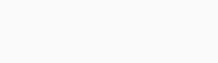As 1 mole i.e., 60 g of cobalt contains 6.023 × 1023 atoms, so, the mass of cobalt required for given rate of disintegration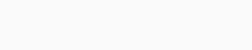Question 10.
The half-life of 9038Sr is 28 years. What is the disintegration rate of 15 mg of this isotope?
Solution:

Question 11.
Obtain approximately the ratio of the nuclear radii of the gold isotope 19779Au and the silver isotope 10747Au.
Solution:
We know the radius of nucleus depend upon mass number ‘A’Question 12.
Find the Q-value and the kinetic energy of the emitted a-particle in the a-decay of
(a) 22688Ra and (b) 22086Rn.
Given m(22688Ra) = 226.02540 u,
m(22286Rn) = 222.01750 u,
m(22086Rn) = 220.01137 u,
m(21684Po) = 216.00189 u, and
mx = 4.00260 u.
Solution:Question 13.
The radionuclide “C decays according to 116C→115B + e+ + v: T1/2 = 20.3 min
The maximum energy of the emitted positron is 0.960 MeV.
Given the mass values:
m(116C) = 11.011434 u
m(115B) = 11.009305 u
Calculate Q and compare it with the maximum energy of the positron emitted.
Solution:
The given equationAs we know that different positrons comes out with different possible energies shared between daughter nucleus and positron.
So, the Q value of reaction is almost same as the maximum energy of positron emitted.

Question 14.
The nucleus 2310Ne decays by β emission. Write t down the β– decay equation and determine
r the maximum kinetic energy of the electrons emitted. Given that:
m(2310Ne) = 22.994466 amu,
m(2311Na) = 22.989770 amu.
Solution:
The β decay of 2310Ne may be explained asAs 2311Na is massive, the kinetic energy released is mainly shared by electron-positron pair. When the neutrino carries no energy, the electron has a maximum kinetic energy equal to 4.374 MeV.

Question 15.
The Q value of a nuclear reaction A + b—>C+d is defined by Q = [mA + mb-mc– md] c2, where the masses refer to the respective nuclei, Determine from the given data the Q-value of the following reactions and state whether the reactions are exothermic or endothermic.
(i) 11H+31H→21H+21H
(ii) 126C+126C→2010C+42C
Atomic masses are given to beSolution:
(i) Let us find the Q value in given first equation,Negative Q value shows that reaction is endothermic.
(ii) Q value in the given second equationPositive Q shows that the reaction is exothermic.

Question 16.
Suppose, we think of fission of a 5626Fe nucleus into two equal fragments, 2813AI. IS the fission energetically possible? Argue by working out Q of the process.
Given, m(5626Fe) = 55.93494 u
and m(2813Ai) = 27.98191 u.
Solution:
The fission of Fe-56 into two fragments ofAs the Q-value is negative, the fission is not possible energycally.

Question 17.
The fission properties of 23994Pu are very similar to those of 23592U. The average energy released per fission is 180 MeV. How much energy, in MeV, is released if all the atoms in 1 kg of pure 23994Pu undergo fission?
Solution:Question 18.
A 1000 MW fission reactor consumes half of its fuel in 5.00 y. How much 23592U did it contain initially? Assume that the reactor operates 80% of the time and that all the energy generated arises from the fission of 23592Uand that this nuclide is consumed by the fission process.
Solution:
In the fission of one nucleus of 23592U, energy generated is 200 MeV.Question 19.
How long can an electric lamp of 100 W be kept glowing by fusion of 2.0 kg of deuterium? Take the fusion reaction as
21H+21H→32He+n+3.27MeV
Solution:
Number of atoms present in 2 g of deuterium = 6.023 × 1023 Total number of atoms present in 2000 g of deuterium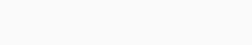Energy released in the fusion of 2 deuterium atoms = 3.27 MeVQuestion 20.
Calculate the height of potential barrier for a head-on collision of two deuterons. The effective radius of deuteron can be taken to be 2fm.
Solution:
For head on collision, distance between centers of two deuteronsThis is a measure of height of coulomb barrier.

Question 21.

From the relation R = R0 A1/3, where R2 is a constant and A is the mass number of a nucleus, show that the nuclear matter density is nearly constant (i.e., independent of A).
Solution:As R is constant, p is contact so, nuclear density is constant irrespective of mass number or size.

Question 22.
For the β+ (positron) emission from a nucleus, there is another competing process known as electron capture (electron from an inner orbit, say, the /(-shell, is captured by the nucleus and a neutrino is emitted).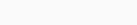Show that if β+ emission is energetically allowed, electron capture is necessarily allowed but not vice-versa.
Solution:
Let us first consider positron emission.This mean if Q1 > 0 then Q2 > 0 but vice vesa is not necessarily allowed. So, electron capture is not necessary for positron emission.

Question 23.
In a periodic table the average atomic mass of magnesium is given as 24.312 u. The average value is based on their relative natural abundance on earth. The three isotopes and their masses are 2412Mg (23.98504 u), 2512Mg (24.98584 u) and 2612Mg (25.98259 u).The natural abundance of 2412Mg 78.99% by mass. Calculate the abundances of the other two isotopes.
Solution:
Let the abundance of isotope 2612Mg isQuestion 24.
The neutron separation energy is defined as the energy required to remove a neutron from the nucleus. Obtain the neutron separation energies of the nuclei 4120Ca and 2713Ai from
the following data:Solution:
Neutron separation of 4020Ca can be obtained as E = Energy equivalent of total mass afterward – Energy equivalent of nucleus beforeThis calculator computes any of the values in the half-life formula given the rest values.

Question 25.
A source contains two phosphorus radio -nuclides 3215P (T1/2 = 14.3 days) and 3315P (Tv2 = 25.3 days). Initially, 10% of the decays come from 3215P How long one must wait until 90% do so?
Solution:
In the mixture of P-32 and P-33 initially 10% decay came from P-33. Hence initially 90% of the mixture is P-32 and 10% of the mixture is P-33. Let after time’t’ the mixture is left with 10% of P-32 and 90% of P-33. Half life of both P-32 and P-33 are given as 14.3 days and 25.3 days respectively. Let V be total mass undecayed initially and ‘y’ be total mass undecayed finally. Let initial number of P-32 nuclides = 0.9 x Final number of P-32 nuclides = 0.1 y Similarly, initial number of P-33 nuclides = 0.l x Final number of P-33 nuclides = 0.9 y For isotope P-32Question 26.
Under certain circumstances, a nucleus can decay by emitting a particle more massive than an a-partide. Consider the following decay processes: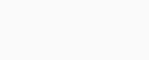Calculate the Q-values for these decays and determine that both are energetically allowed.
Solution:
Let us calculate Q value for the given decay process. For first decay processSince, Q value is positive in both the cases, hence decay process in both ways are possible

Question 27.
Consider the fission of 23892U by fast neutrons. In one fission event, no neutrons are emitted and the final end products, after the beta decay of the primary fragments are 14058Ce and 3215Ru. Calculate Qfor this fission process. The relevant atomic and particle masses are:Solution:
The fission of U-238 by fast neutrons into fragments Ce-140 and Ru-99 with energy released Q can be written asQuestion 28.
Consider the D-T reaction (deuterium – tritium fusion)
21H+31H→42He+n
(a) Calculate the energy released in MeV in this reaction from the data
m (21H) = 2.014102 u, m (31H) = 3.016049 u
(b) Consider the radius of both deuterium and tritium to be approximately 2.0 fm. What is the kinetic energy needed to overcome the coulomb repulsion between the two nuclei? To what temperature must the gases be heated to initiate the reaction?
Solution:

Classically, K.E. atleast equal to this amount is required to overcome Coulomb repulsion. Using the relationQuestion 29.
Obtain the maximum kinetic energy of β-particles and the radiation frequencies of y-decays in the decay scheme shown in figure. You are given that
m(19879Au) = 197.968233 u, m(19880Ag) = 197.966760 uSolution:
Energy corresponding to y1Question 30.
Calculate and compare the energy released by
(a) fusion of 1.0 kg of hydrogen deep within the Sun and
(b) the fission of 1.0 kg of 235U in a fission reactor.
Solution:
(a) In the fusion reactions taking place within core of sun, 4 hydrogen nuclei combines to form a helium nucleus with the release of 26 MeV of energy.(b) Energy released per fission of U-235 is 200 MeV.So the energy released in fusion of 1 kg of Hydrogen is nearly 8 times the energy released in fission of 1 kg of uranium-235.

Question 31.
Suppose India has a target of producing by 2020 AD, 200,000 MW of electric power, ten percent of which is to be obtained from nuclear power plants. Suppose we are given that, on an average, the efficiency of utilization (/.e., conversion to electric energy) of thermal energy produced in a reactor was 25%. How much amount of fissionable uranium would our country need per year? Take the heat energy per fission of 235U to be about 200 MeV.
Solution:
10% of total power 200,000 MW to be obtained from nuclear power plant by 2020 AD.Hence mass of uranium needed per year = 3.08 × 104 kg